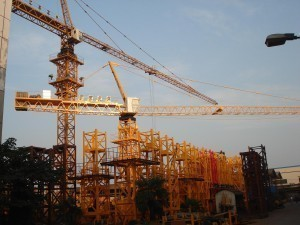# Crane Dimensions

There are many types of cranes, including carriermounted, rough terrain etc. The models below pertain to all-terrain cranes.

### Crane Dimensions: Grove

For the GMK2035 model, the rated lift capacity is 35 tons at a 10 ‘ radius. The maximum boom lift is 105′ 0" and the max jib tip ht is 152’ 6". The main hoist line pull is 7419 lbs and the engine gross power is 275 hp. Its weight is 52910 lbs.

The GMK6250-L has a rated lift capacity of 250, and a max boom tip ht of 246′ 0". The jib tip ht is 341′ 0". The hoist line pull is 21000 lbs and the engine gross power is 525 hp. The weight is 133900 lbs.

### Crane Dimensions: Liebherr

The model LTC 1055-3.1 has a rated lift capacity of 55 tons at 10′ radius. The maximum hoist line is 10116 lbs and the weight is 79200 lbs. The LTM 1030-2.1 crane has a rated lift of 42 tons and a main hoist line pull of 7400 lbs. The engine gross power is 278 hp and the weight is 52800 lbs.

Model LTM 1800 has a rated lift of 882 tons with a jib tip ht of 479′ 0". The main hoist line pull is 31460 lbs. The engine gross power is 598 hp and the weight is 211641 lbs. The LTM 1500-8.1 crane has a rated lift of 595, a main hoist line pull of 28350 lbs and engine gross power of 598 hp. it weighs 211640 lbs.

Model ATF-1500XL has a rated lift capacity of 150 tons (10′ radius) with a maximum boom tip ht of 174′ 0". The maximum jib tip ht is 271’ and the main hoist line pull is 18650 lbs. The engine gross power is 503 hp. The weight is 197000 lbs.

The ATF-650XL’s rated lift capacity is 65 tons and the maximum boom tip ht is 140′ 0". The jib tip ht is 191′ 0". The main hoist line pull is 10000 lbs and the weight is 109250 lbs. The engine hp is 349.

### Crane Dimensions: Terex

The ATT 1354 model has a rated lift capacity of 130 (10′ radius) with a main hoist line pull of 18800 lbs. Its weight is 116800 lbs and the engine power is 435 hp. The ATT 900’s lift capacity is 90. The main hoist line pull is 17700 lbs and the weight is 113600 lbs.

The engine is 428 hp. The ATT 600-4 model has a 60 ton lift and the main hoist line pull is 12346 lbs. The engine power is 313 hp and the weight is 105400 lbs. The ATT 400/2 has a rated lift capacity of 44 tons and the boom tip ht is 103′ 8". The jib tip ht is 154′ 2". The weight is 51000 lbs. The engine power is 279 hp.

When looking at crane dimensions, always check your needs and requirements. This will determine the type you’ll want to employ. If you’re not sure, consult a building / construction expert.